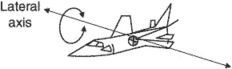# lateral axis

## lateral axis

(naval architecture)
The athwartship line approximately through the center of gravity of a craft, around which it pitches.

## lateral axisA straight line through the center of gravity that is parallel to the line joining the wing tips. The aircraft pitches around this axis. See axes.
References in periodicals archive ?
When he did, he learned that the correct pivotal altitude for a given groundspeed allows a banked line of sight from the cockpit directly parallel to the lateral axis of the aircraft to the pylon, a stationary object on the ground.
However, more sophistication is needed to resolve the tensions between distinguishing the vertical axis from the lateral axis (Mitchell, 2003).
[F.sub.[SIGMA]x] is the sum of all forces projected on the longitudinal axis and [F.sub.[SIGMA]y] is the sum of all forces projected on the lateral axis. [M.sub.[SIGMA]] is the sum of moments.
Asymptotic gain is [K.sub.A]/M[s.sup.2]; the asymptote intersects with the lateral axis at frequency [[omega].sub.s], [[omega].sub.s] = [square root of [K.sub.A]/M]; (9) can be written as
In general, this model should be considered in the unbounded region Q = [Q.sub.z,x] x (0, T], where [Q.sub.z,x] = {(z, x) : (z, x) [member of] (0, L) x R} is the spatial domain, L represents the length of the device, x is the coordinate of the unbounded lateral axis of the device, and T defines the length of the time interval where we perform the integration.
Specifically, it modifies a foot angle about the roll axis and a foot-landing point along the lateral axis so that a swing foot contacts the ground horizontally.
The drawbacks are, they require the most horsepower to plane off, draft the most water and roll on their lateral axis at rest.
Rotation, about a vertical or lateral axis, causes the brain to twist within the skull, impacting the bone and bruising the tissue.
(9) If we take, however, the idea of bodies in relation to other bodies, then this chain of being produces a lateral axis wherein social configurations that are in relation to each other are also present.
For example, in TID descriptions, two objects located on a lateral axis can be represented either on the left-right axis or the front-back axis in the signing space.
The researchers discovered that if a string was threaded through the central hole and then stretched tight to make the disc rotate about its lateral axis, the result was a superimposition of the two pictures on the retina.
In the case of one engine's failure, compensation of pitching movements which can be resulted in continuous altitude change or periodical rotation about the lateral axis occures.

Site: Follow: Share:
Open / Close